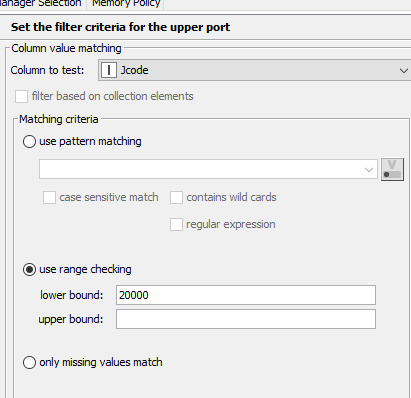# Evaluate column value with Column Expressions

I have a file where I am trying to create two new columns. In this example, I need a new column of data with just Hourly rates and another new column with Annual rates. The values of the new columns will be derived from the Salary column, so I need to convert some of the data into hourly rates and other data to Annualized rates. The Jcode column determines what data should be in what column. Jcode below 20000 indicates jobs that should be written to a Hourly column and Jcode of 20000 or higher will be listed in Annual column. In the example below, Row0 Salary should be written to a new column with the rate of 25.00, Row1 will be written as 25.00, Row2 will be written to the new Annual column as 52000, and Row3 will be written in the new Annual column as 52000.

I had envisioned using the Column Expressions node, but am unfamiliar with syntax of writing that logic. Any suggestions on how to accomplish this?Your description and image do not really line-up because based on your logic (< 20000) the first two should be hourly and the last two should be annual right?

You can achieve this with a Column Expression yes, with two expressions:

Salary:

``````if (column("Jcode") < 20000) {
25
} else {
52000
}
``````

Rate:

``````if (column("Jcode") < 20000) {
"Hourly"
} else {
"Annual"
}
``````

Alternatively, you can use a Java Snippet to output as many columns as you like under the same if clause.

``````if (c_Jcode < 20000) {
out_Salary = 25;
out_Rate = "Hourly";
} else {
out_Salary = 52000;
out_Rate = "Annual";
}
``````

Hope this helps!

1 Like

I think this is getting closer, but in rows where the data in the Salary column is reported as an annual rate but falls within a hourly Jcode, I need to perform math operation to convert it to an hourly rate. So for example if the rate was entered as \$40,000, I would divide it by 2080 (i.e., # of hours) to return \$19.23. Conversely, if data in the Salary column was reported as \$52000 but it fell within a hourly Jcode, I would divide the annual rate by 2080 to get \$25. The Rate column should indicate if the values are Hourly or Annual.

I tried converting your example, but it does not appear to take into account what the original rate was.

if (column(“Jcode”) < 20000) {
column(“Salary”)/2080
} else {
column(“Salary”)
}

Your situation is a bit confusing to me.

Here you mention that you only have to he jobcode and the rest should created.

This assumes that the other columns are already there. Am I right that actually Salary and Rate both need to be evaluated to determine if they are in the correct dimension based on the Jcode and, if incorrect, should be adjusted?

Something like this will take the Rate into account.

Salary (change the output from int to double):

``````if (column("Jcode") < 20000 && column("Rate").equals("Annual")) {
column("Salary") / 2080
} else if (column("Jcode") > 20000 && column("Rate").equals("Hourly")) {
column("Salary") * 2080
} else {
column("Salary")
}
``````

Rate:

``````if (column("Jcode") < 20000 && column("Rate").equals("Annual")) {
"Hourly"
} else if (column("Jcode") > 20000 && column("Rate").equals("Hourly")) {
"Annual"
} else {
column("Rate")
}
``````

Output:1 Like

That worked nicely. Thanks so much for your assistance. I appreciate it.

1 Like

At the risk of going to the well again, I have one more question on this task. How can I create new columns that show just the Hourly rates and another new column that show just the Annualized rates. My current attempt has was using the Row Splitter node and then rejoining with a Joiner node but that is not bearing fruit. I can successfully split the data, but joining with the seperate columns is proving challenging. Any ideas would be greatly appreciated.That’s something for the concat node.

This topic was automatically closed 7 days after the last reply. New replies are no longer allowed.